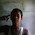### Program 218:One Time Pad Cipher using C

Program 218: To understand What is One Time Pad Cipher ?

```#include<stdio.h>
#include<string.h>
#include<ctype.h>
main()
{
//All the text which ever entered is converted to upper and without spaces
int i,j,len1,len2,numstr,numkey,numcipher;
char str,key,cipher;
printf("Enter a string text to encrypt\n");
gets(str);
for(i=0,j=0;i<strlen(str);i++)
{
if(str[i]!=' ')
{
str[j]=toupper(str[i]);
j++;
}
}
str[j]='\0';
//obtaining numerical plain text ex A-0,B-1,C-2
for(i=0;i<strlen(str);i++)
{
numstr[i]=str[i]-'A';
}
printf("Enter key string of random text\n");
gets(key);
for(i=0,j=0;i<strlen(key);i++)
{
if(key[i]!=' ')
{
key[j]=toupper(key[i]);
j++;
}
}
key[j]='\0';
//obtaining numerical one time pad(OTP) or key
for(i=0;i<strlen(key);i++)
{
numkey[i]=key[i]-'A';
}

for(i=0;i<strlen(str);i++)
{
numcipher[i]=numstr[i]+numkey[i];
}
//To loop the number within 25 i.e if addition of numstr and numkey is 27 then numcipher should be 1
for(i=0;i<strlen(str);i++)
{
if(numcipher[i]>25)
{
numcipher[i]=numcipher[i]-26;
}
}
printf("One Time Pad Cipher text is\n");
for(i=0;i<strlen(str);i++)
{
printf("%c",(numcipher[i]+'A'));
}
printf("\n");

}

```
Explanation:
Pre Explanation:

//Coming Soon...

Output:

1.there is code for decrypt?
I,m still newbie
thank u

1.2.thank you, it helps me a lot,
in OTP algorithm, can we encypt with 255 characters?

3.Dude, if you use "gets" as a function you kind of defeat the "perfect security" purpose, i know that this is probably not meant to be used professionally but using gets really exposes your code to a buffer overflow, it's a very bad practice in general.

1.https://stackoverflow.com/questions/1694036/why-is-the-gets-function-so-dangerous-that-it-should-not-be-used
here's a link on why you should forget gets existence

4.your program is very efficient.it have much help me.

5.Bitcoin money is a cryptocurrency fork of Bitcoin exemplary. It was made in august 2017. It is significant that Bitcoin money builds the size of squares, which permits more exchanges to be prepared. bitcoin mixer

 Donate Buy me a coffee \$2.00 USD Buy me a burger \$5.00 USD Buy me a pizza \$10.00 USDCompiler Used by me C-Free 5.0(Recommended) Other Compilers Code Blocks(Recommended) Online Compilers Ideone(Remember to give input before executing online where ever necessary)